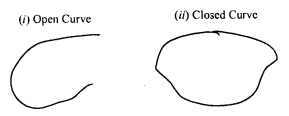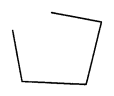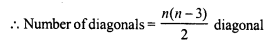# RD Sharma Class 8 Solutions Chapter 15 Understanding Shapes I Ex 15.1

In this chapter, we provide RD Sharma Class 8 Solutions Chapter 15 Understanding Shapes I Ex 15.1 for English medium students, Which will very helpful for every student in their exams. Students can download the latest RD Sharma Class 8 Solutions Chapter 15 Understanding Shapes I Ex 15.1 Maths pdf, free RD Sharma Class 8 Solutions Chapter 15 Understanding Shapes I Ex 15.1 book pdf download. Now you will get step by step solution to each question.

### RD Sharma Solutions for Class 8 Chapter 15 Understanding Shapes I Ex 15.1Download PDF

Question 1.
Draw rough diagrams to illustrate the following:
(i) Open curve
(ii) Closed curve
Solution:Question 2.
Classify the following curves as open or closed.Solution:
Open curves : (i), (iv) and (v) are open curves.
(ii) , (iii), and (vi) are closed curves

Question 3.
Draw a polygon and shade its interior. Also draw its diagonals, if any.
Solution:
In the given polygon, the shaded portion is its interior region AC and BD are the diagonals of polygon ABCD.Question 4.
Illustrate, if possible, each one of the following with a rough diagram:
(i) A closed curve that is not a polygon.
(ii) An open curve made up entirely of line segments.
(iii) A polygon with two sides.
Solution:
(i) Close curve but not a polygon.(ii) An open curve made up entirely of line segments.(iii) A polygon with two sides. It is not possible. At least three sides are necessary

Question 5.
Following are some figures : Classify each of these figures on the basis of the following:(i) Simple curve
(ii) Simple closed curve
(iii) Polygon
(iv) Convex polygon
(v) Concave polygon
(vi) Not a curve
Solution:
(i) It is a simple closed curve and a concave polygon.
(ii) It is a simple closed curve and convex polygon.
(iii) It is neither a curve nor polygon.
(iv) it is neither a curve not a polygon.
(v) It is a simple closed curve but not a polygon.
(vi) It is a simple closed curve but not a polygon.
(vii) It is a simple closed curve but not a polygon.
(viii) It is a simple closed curve but not a polygon

Question 6.
How many diagonals does each of the following have ?
(ii) A regular hexagon
(iii) A triangle.
Solution:
Here n = 4Question 7.
What is a regular polygon ? State the name of a regular polygon of:
(i) 3 sides
(ii) 4 sides
(iii) 6 sides.
Solution:
A regular polygon is a polygon which has all its sides equal and so all angles are equal,
(i) 3 sides : It is an equilateral triangle.
(ii) 4 sides : It is a square.
(iii) 6 sides : It is a hexagon.

All Chapter RD Sharma Solutions For Class 8 Maths

—————————————————————————–

All Subject NCERT Exemplar Problems Solutions For Class 8

All Subject NCERT Solutions For Class 8

*************************************************

I think you got complete solutions for this chapter. If You have any queries regarding this chapter, please comment on the below section our subject teacher will answer you. We tried our best to give complete solutions so you got good marks in your exam.

If these solutions have helped you, you can also share rdsharmasolutions.in to your friends.